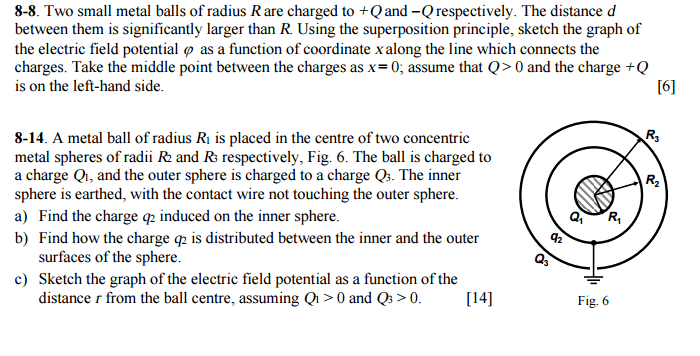# Grounded conductor and Electric field potential question ?

• Karamany

## Homework Statement[/B]

V= kq/r.[/B]

## The Attempt at a Solution

For 8-8, I do not really know how to approach it.
For 8-14 , I think that q2=-q1 and q3=q1+q2.
I can now use V=kq/r and then find the equations for r>R3 , r=R3 , R2<r<R3 , r=R2 and R1<r<R2 and then graph. Am I right?

For 8-8 - the question tells you how to approach it.
Sketch the situation, then, using a dotted line, sketch the potentials for each ball separately.
Then apply the superposition principle - draw in the result as a bold line.

For 8-14 - please show your reasoning about the charges, ... ie. what happens to the charge distribution on sphere 2 and why?

## Homework Statement

View attachment 110285[/B]

8-14: the "spheres" quoted in the problem are actually shells. There is a central solid sphere or ball of radius R1, then infinitely thin shells of radii R2 and R3.

There are thus 5 surfaces with respective charge densities (from innermost to outermost): σ1, σ2, σ3, σ4 and σ5.
σ1 should be obvious. From that, σ2 should be readily calculated.
That leaves σ3, σ4 and σ5. You can get 3 independent equations in these 3 unknowns. One involving σ4 and σ5 is obvious. One involving σ3 and σ4 should also be apparent; similar idea to σ1 and σ2. For the last equation, force zero E field inside the grounded shell.
Having all 5 surface charge densities enables you to compute everything else.
For 8-14 , I think that q2=-q1 and q3=q1+q2.
I can now use V=kq/r and then find the equations for r>R3 , r=R3 , R2<r<R3 , r=R2 and R1<r<R2 and then graph. Am I right?
I don't think so. For one thing that would make q3 = 0.